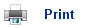# Rates of Chemical Reactions

• All chemical reactions have different rates of reactions.
• The rate of a chemical reaction can be increased by:
• Increasing the concentration of a solution.
• Raising the pressure of a gas.
• Increasing the temperature of the reaction.
• Increasing the surface area of a solid.

## The effect of changing the concentration

• An example often used when demonstrating chemical reactions is the reaction between hydrochloric acid and calcium carbonate:

CaCO3(s) + 2HCl(aq)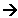CaCl2(s) + H2O(l) + CO2(g)

• The rate of reaction can be altered by changing the size of the calcium carbonate chips or the concentration of the acid.
• If the chips are large and in excess, then only a small fraction of the solid reacts and the chips don’t change shape. This means that the calcium carbonate does not affect the rate of reaction, only the concentration of the hydrochloric acid does.
• The rate will decrease over time as the hydrochloric acid is used up (its concentration decreases).
• The rate of reaction could be worked out either by measuring the pH of the solution, the decrease in the mass of calcium carbonate, or the increase in the volume of carbon dioxide.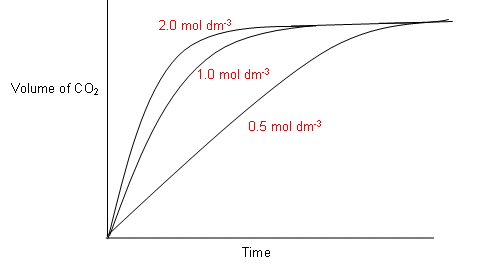• The graph above shows the volume of gas produced over time in the reaction between HCl and CaCO3.
• Each reaction started with 2 mol of HCl with excess calcium carbonate. As you can see from the graph, the rate of reaction tails off towards the end, as the HCl is being used up, i.e. its concentration is decreasing.
• All three reactions result in the same amount of CO2 gas being produced; however the higher the concentration of HCl, the faster the rate of reaction, thus the less time it takes for the reaction to be complete.

## Rate Equations

• As can be seen from the previous graphs, the rate is constant at first (i.e. the graphs are linear for around the first 20% of the total reaction). This initial stage of the reaction can be used to measure the initial rate of reaction.
• The table below shows the time taken to produce 50cm3 of CO2 for each concentration of HCl: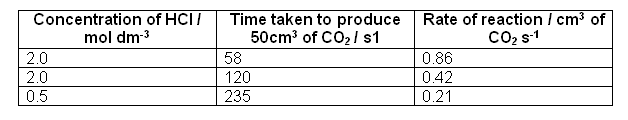• The units of the rate above are measured in cm3 of CO2 per second; this is because it is how the rate of reaction has been measured. If the rate was measured using the decrease in concentration of HCl instead, the units of rate would be mol dm-3 s-1, or if it was measured by decrease in mass it would be g s-1.
• From the results in the table above, it can be worked out that the rate of reaction is directionally proportional to the concentration of hydrochloric acid, so if you halve the concentration of HCl will be halved, if you quarter the concentration, the rate will be quartered.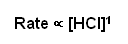• This relationship can be converted into a rate equation by replacing the proportionality sign with an equals sign and inserting a constant:

Rate = k[HCl]1

• k is the rate constant.
• If we are measuring the rate of the reaction using the change in concentration, then the units will be: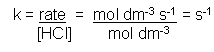• The rate is measured in s-1 in the case above, but in other rate equations it may be different; it all depends on the units being used to measure the rate and the number of reactants and products.

## Orders of Reactions

• The reaction of calcium carbonate with hydrochloric acid is said to be first order with respect to hydrochloric acid.
• This is because the rate depends upon the concentration of hydrochloric acid to the power one.
• Take the rate equation below:

Rate = k[A]n[B]m

• n is the order of the reaction with respect to A and m is the order of reaction with respect to B.
• The values of n and m are usually 0, 1 or 2 corresponding to zero, first and second orders; however some reactions can have fractional or negative orders.
• Orders of reactions must be worked out experimentally; they are totally unrelated to the chemical equation.

## Working out a rate equation

• An experiment was conducted to find out how the concentration of X and Y affect the rate of formation of the product Z. the results were as follows:• Firstly to work out the order of the reaction. Starting with X, we need to look in the table for two different values of [X], when the concentration of Y is kept constant. Experiments 2 and 3 can be used to work out the order of X.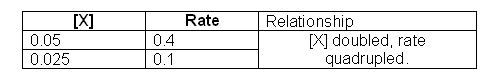• When [X] is doubled the rate quadruples, this means that the order of the reaction with respect to [X] is 2.
• Now to work out the order of the reaction with respect to X, in this case we need to use reaction 1 and 2: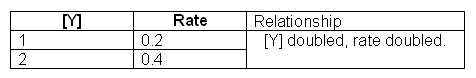• The order of this reaction with respect to Y is one, as the relationship between [Y] and rate is directionally proportional.
• The rate equation for the reaction is:

Rate = k[X]2[Y]

• The overall order of the reaction is three (the two orders added together).
• Now to work out the constant.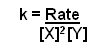• This can be worked out by using values from the table: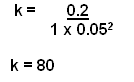• Note that this k value is only applicable for one temperature.
• The overall reaction is

Rate = 80[X]2[Y]

• Now for the units: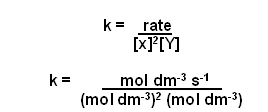• Cancelling down gives mol-2 dm6 s-1.

## Half-Lives

• When analysing a rate graph, it is possible to look at the initial rate of the reaction to work out the order (as done previously); however it is also possible to look at the half-life for a first order reaction.
• The half-life is the time taken for the concentration of a substance to fall to half its starting value. It is often given the symbol t/2.
• The reaction of calcium carbonate with hydrochloric acid (looked at previously) can be used to analyse half-lives:

CaCO3(s) + 2HCl(aq)CaCl2(s) + H2O(l) + CO2(g)

• The volume of CO2 produced over time is given in the following graph: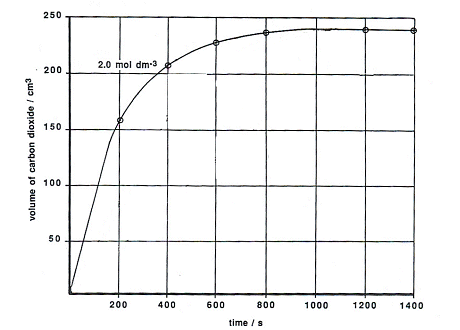• Looking at the graph, the reaction is half completed when the concentration produced is 120 cm3, at this point the concentration of HCl is 1 mol dm-3. The time taken to reach this point is the half-life of the reaction with a starting concentration of 2 mol dm-3. The half-life for this is 141 seconds.
• The time taken for the volume of CO2 to change from 120 cm3 to 180 cm3 corresponds to a change in concentration of HCl from 1 mol dm-3 to 0.5 mol dm-3, i.e. it is the t/2 value for the reaction with a starting concentration of 1 mol dm-3. The half-life for this is 144 seconds.
• The next half-life can be found by calculating the time it takes for the volume of CO2 to increase from 180cm3 to 210cm3. This corresponds to [HCl] falling from 0.5 mol dm-3 to 0.25 mol dm-3. The half-life for this is 147 seconds.
• The half-lives for the three different parts of the equation are roughly the same; they are independent of the starting concentration of HCl. Therefore the reaction is first order with respect to [HCl].
• Second and zero order processes do not have constant half-lives.
• First order processes are never over, the mass is constantly halving every t years. A reaction can be classed as over when the change can no longer be measured.

## Rate Determining Steps

• As previously mentioned, the rate of a chemical reaction cannot be worked out by looking at the chemical equation.
• This is because most reactions do not take place in one step, but in many. The chemical equation only shows the overall effect of these many small steps.
• For example, the reaction below shows the nucleophilic substitution reaction of 2-bromo-2-methylpropane with OH-: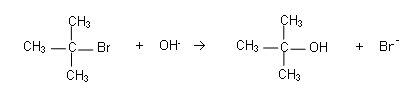• The reaction is first order with respect to C(CH3)3Br and zero order with respect to OH-:

Rate = k[C(CH)3Br]

• The [OH-] is not involved in the rate equation, i.e. altering it does not have an effect upon the rate of the reaction.
• The reason for this is that the reaction does not occur in one step, but it occurs in two steps:
1. The C-Br bond breaks heterolytically: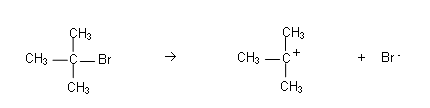This only involves C(CH3)3Br, thus its rate is only dependent on [C(CH3)3Br].
2. The carbocation reacts with OH-: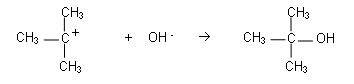The reaction for step 2 is much faster than the reaction in step 1. The rate of reaction is always determined by the slowest reaction, therefore it is reaction 1 that is the rate determining step and its equation becomes the rate equation for the whole reaction.

## Useful books for revision

Revise A2 Chemistry for Salters (OCR A Level Chemistry B)Salters (OCR) Revise A2 ChemistryHome